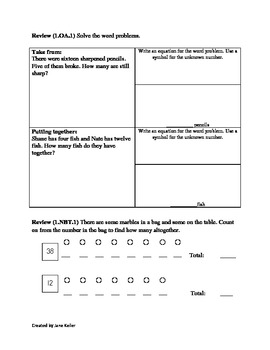# 1st Grade Common Core Math Worksheets 1.OA.3 Addition and Subtraction StrategiesSubject
Grade Levels
Resource Type
Product Rating
File Type

PDF (Acrobat) Document File

Be sure that you have an application to open this file type before downloading and/or purchasing.

166 KB|10 pages
Share
Product Description
1st Grade Common Core Word Problem Worksheets 1.OA.3 Addition and Subtraction Strategies

The attachment is a packet of five days of worksheets for first grade common core standard CCSS.Math.Content.1.OA.B.3 Apply properties of operations as strategies to add and subtract.2 Examples: If 8 + 3 = 11 is known, then 3 + 8 = 11 is also known. (Commutative property of addition.) To add 2 + 6 + 4, the second two numbers can be added to make a ten, so 2 + 6 + 4 = 2 + 10 = 12. (Associative property of addition.)

Each day has two worksheets, designed to be copied front and back. The front page focuses on the standard of the week and the back page is a review of previous information. Each sheet contains place value review and word problems, including an area to write an equation when applicable.

This worksheet was designed to be the first nine weeks of school and there are no missing addend or subtrahend problems.

The review standards are:

CCSS.Math.Content.1.OA.A.1 Use addition and subtraction within 20 to solve word problems involving situations of adding to, taking from, putting together, taking apart, and comparing, with unknowns in all positions, e.g., by using objects, drawings, and equations with a symbol for the unknown number to represent the problem.

CCSS.Math.Content.1.NBT.A.1 Count to 120, starting at any number less than 120. In this range, read and write numerals and represent a number of objects with a written numeral.

Total Pages
10 pages
Answer Key
N/A
Teaching Duration
1 Week
Report this ResourceSign Up